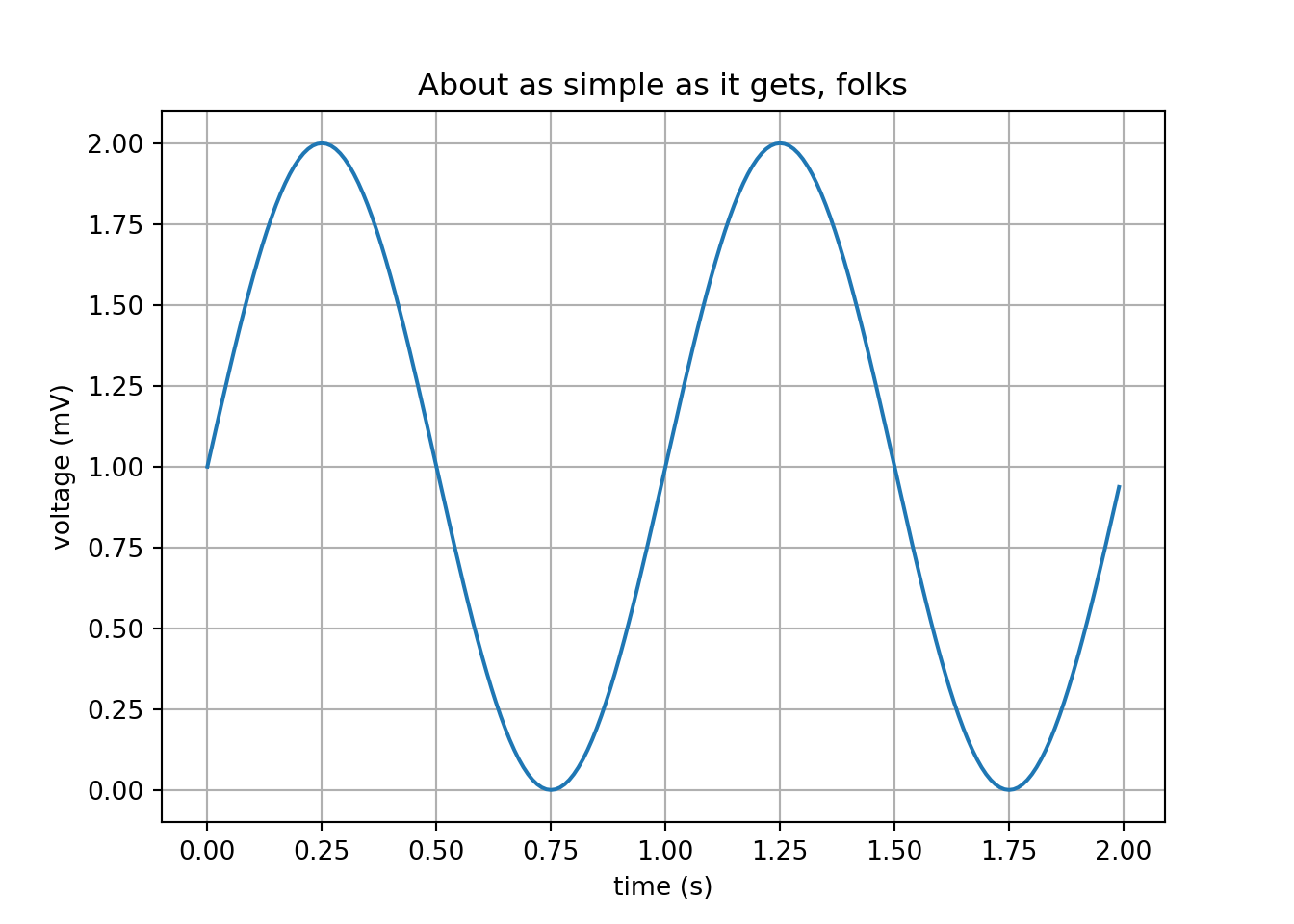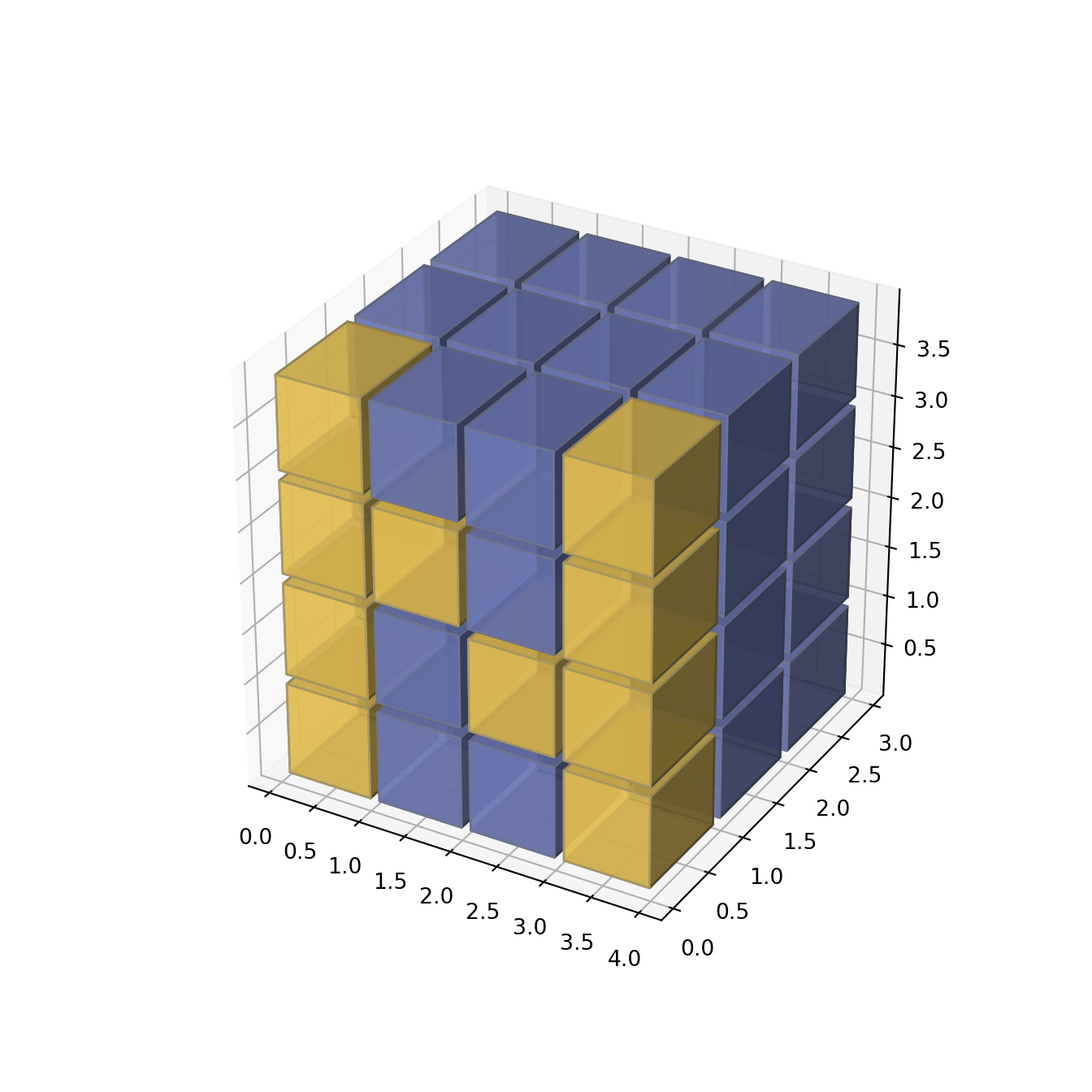Why I decided to publish data science in a blog

Alfonso R. Reyes
(7 January 2019)

OnlineIntroduction

As I announced last week, my blog is now online at http://blog.oilgainsanalytics.com. LinkedIn may obfuscate the link so I am also providing it as an image below. Clicking on the image will bring you to the blog:

blog.oilgainsanalytics.com

or

oilgainsanalytics.com/blog

Motivation

I believe in the sharing philosophy of data science as I learned it from my biostatistician instructors at Johns Hopkins University (Peng, Leek, Caffo, et al). There are so many career-changing lessons that you could pick up with these professors at Coursera. Data Science is a whole multidisciplinary, or should I say interdisciplinary as in Wikipedia, field that combines statistics, mathematics, computer science, and data engineering that gives you the power to dig deep into your data and make astonishing discoveries to bring real value to your organization.

I felt the need of an online website that could support Rmarkdown instead of copying and pasting code and pictures to [LinkedIn] every time.

Under the hood

This blog uses the R package blogdown, an application that converts markdown and Rmarkdown to Html and Javascript, making easier for me to run code and publish it immediately as it exactly is written.

One of the articles I am preparing on `multiwell statistics` -coming in few days-, will be written in Python and R together using RStudio IDE1. It will be about running statistical methods on datasets originated by batch automation produced by communicating with the production optimization software Prosper via OpenServer, work that I developed few years ago but very relevant nowadays.

Still, I will continue publishing in LinkedIn but the extended, best and more current version of the post running with R and Python code will be in the blog.

A quick demonstration

This first example assumes you installed a Portable Python WinPython 3.7.1, 64-bit, under your user directory in Windows. Not to worry, I will explain in a separate set of slides how to get Python installed.

First, we load a Python environment. In this case, I am loading the Python portable version I mentioned above, but it could be Anaconda or another flavor. I have found that WinPython is just much easier to navigate and make it work.

This is the code that load the Pythobn environment that will together with R.

``````# load the package that makes R and Python talk
library(reticulate)

# set the preferred Python to execute
user_profile <- Sys.getenv("USERPROFILE")                    # user folder
python_portable <- normalizePath(file.path(user_profile,     # Python location
"WPy-3710zero/python-3.7.1.amd64/python.exe"))

reticulate::use_python(python_portable, required = TRUE)

# find out if it took it
reticulate::py_config()
#> python:         C:\Users\msfz751\WPy-3710zero\python-3.7.1.amd64\python.exe
#> libpython:      C:/Users/msfz751/WPy-3710zero/python-3.7.1.amd64/python37.dll
#> pythonhome:     C:\Users\msfz751\WPY-37~1\PYTHON~1.AMD
#> version:        3.7.1 (v3.7.1:260ec2c36a, Oct 20 2018, 14:57:15) [MSC v.1915 64 bit (AMD64)]
#> Architecture:   64bit
#> numpy:          C:\Users\msfz751\WPY-37~1\PYTHON~1.AMD\lib\site-packages\numpy
#> numpy_version:  1.15.4
#>
#> NOTE: Python version was forced by use_python function``````

Example 1

Produce a simple Python matplotlib plot.

This is not easy as it seems using other flavors of Python. I found that WinPython makes this plotting task easier for beginners or advanced levels. But this should be your test of fire because it uses several Python packages: `matplotlib`, `numpy`, `PyQt`, etc. So, if this works, almost everything else will work.

``````# a simple sine plot
import matplotlib.pyplot as plt
import numpy as np
t = np.arange(0.0, 2.0, 0.01)
s = 1 + np.sin(2*np.pi*t)
plt.plot(t, s)
plt.xlabel('time (s)')
plt.ylabel('voltage (mV)')
plt.title('About as simple as it gets, folks')
plt.grid(True)
plt.savefig("sine.png")
plt.show()``````I know. This can be done easily in Jupyter notebooks but the objective here is to mix the power of Python and R running in a native R platform. I will explain later why.

Why the Python plots if R has `ggplot2`

One big reason: doing some data science and machine learning with reservoir blocks will require some 3d capabilities and Python has packages available for that. R also does but it would take more work. Refer the next example.

Example 2

Now, we are going to plot a pretended reservoir block for reservoir simulation. We are going to need it at sometime to build the machine learning algorithm and a artificial intelligence agent to do, let’s say, history matching, for starters. I found this beautiful example in the `matplotlib` tutorial section.

``````# https://matplotlib.org/api/_as_gen/mpl_toolkits.mplot3d.axes3d.Axes3D.html
#=======================================================
#3D voxel / volumetric plot with cylindrical coordinates
#=======================================================
# Demonstrates using the ``x, y, z`` arguments of ``ax.voxels``.
import matplotlib.pyplot as plt
import matplotlib.colors
import numpy as np
# This import registers the 3D projection, but is otherwise unused.
from mpl_toolkits.mplot3d import Axes3D  # noqa: F401 unused import
def midpoints(x):
sl = ()
for i in range(x.ndim):
x = (x[sl + np.index_exp[:-1]] + x[sl + np.index_exp[1:]]) / 2.0
sl += np.index_exp[:]
return x
# prepare some coordinates, and attach rgb values to each
r, theta, z = np.mgrid[0:1:11j, 0:np.pi*2:25j, -0.5:0.5:11j]
x = r*np.cos(theta)
y = r*np.sin(theta)
rc, thetac, zc = midpoints(r), midpoints(theta), midpoints(z)
# define a wobbly torus about [0.7, *, 0]
sphere = (rc - 0.7)**2 + (zc + 0.2*np.cos(thetac*2))**2 < 0.2**2
# combine the color components
hsv = np.zeros(sphere.shape + (3,))
hsv[..., 0] = thetac / (np.pi*2)
hsv[..., 1] = rc
hsv[..., 2] = zc + 0.5
colors = matplotlib.colors.hsv_to_rgb(hsv)
# and plot everything
fig = plt.figure()
ax = fig.gca(projection='3d')
ax.voxels(x, y, z, sphere,
facecolors=colors,
edgecolors=np.clip(2*colors - 0.5, 0, 1),  # brighter
linewidth=0.5)
plt.show()``````Those blocks are called voxels. If you have seen reservoir simulation you know what they represent. We could also have plot this in cartesian coordinates instead of cylindrical.

Example 3

This is a simpler example of the blocks in cylindrical coordinates above.

Why is this important?

If we will be applying machine learning algorithms we need to have some tools outside the conventional reservoir simulators that do not have machine learning or artificial intelligence capabilities. This means, if you have the reservoir grid plus the saturations, permeability and wells location, it very likely they will be in text readable format. At least, in Eclipse they are. Then, you will able to transform the grid and reservoir properties using your production history and achieve more accurate and faster matching. There is abundant literature on the subject in the SPE papers.

``````# https://matplotlib.org/gallery/mplot3d/voxels_numpy_logo.html
import matplotlib.pyplot as plt
import numpy as np
# This import registers the 3D projection, but is otherwise unused.
from mpl_toolkits.mplot3d import Axes3D  # noqa: F401 unused import
def explode(data):
size = np.array(data.shape)*2
data_e = np.zeros(size - 1, dtype=data.dtype)
data_e[::2, ::2, ::2] = data
return data_e
# build up the numpy logo
n_voxels = np.zeros((4, 3, 4), dtype=bool)
n_voxels[0, 0, :] = True
n_voxels[-1, 0, :] = True
n_voxels[1, 0, 2] = True
n_voxels[2, 0, 1] = True
facecolors = np.where(n_voxels, '#FFD65DC0', '#7A88CCC0')
edgecolors = np.where(n_voxels, '#BFAB6E', '#7D84A6')
filled = np.ones(n_voxels.shape)
# upscale the above voxel image, leaving gaps
filled_2 = explode(filled)
fcolors_2 = explode(facecolors)
ecolors_2 = explode(edgecolors)
# Shrink the gaps
x, y, z = np.indices(np.array(filled_2.shape) + 1).astype(float) // 2
x[0::2, :, :] += 0.05
y[:, 0::2, :] += 0.05
z[:, :, 0::2] += 0.05
x[1::2, :, :] += 0.95
y[:, 1::2, :] += 0.95
z[:, :, 1::2] += 0.95
fig = plt.figure()
ax = fig.gca(projection='3d')
ax.voxels(x, y, z, filled_2, facecolors=fcolors_2, edgecolors=ecolors_2)
plt.show()``````Why Python and R?

I know what you are thinking. If Python has all these plotting capabilities, why not doing all the coding just with Python?

Because:

• R is much better and faster at data manipulation
• In R is easier to use C, C++ and Fortran libraries for matrix and array operations
• Report generation in R is publishing quality.
• You can use embedded Latex, Beamer, tikz, and innumerable packages for equations, presentations, diagrams that belong to the universe of reproducibility in data science.

And the best reason of all: We will be ahead of the pack by using both powerful scripting languages: Python and R.

And with the Volve datasets now available the task will be more productive.

Warning

This page is dynamic, it will be changing in the next hours until the edition is complete. In the meantime, enjoy.

Code

All the code will be available in GitHub at https://github.com/f0nzie/

1. Integrated Development Environment